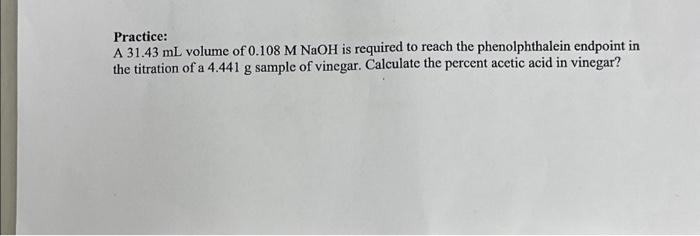Home / Expert Answers / Chemistry / practice-a-31-43-ml-volume-of-0-108-m-naoh-is-required-to-reach-the-phenolphthalein-endpoint-in-the-pa746

# (Solved): Practice: A 31.43 mL volume of 0.108 M NaOH is required to reach the phenolphthalein endpoint in the ...

Practice: A 31.43 mL volume of 0.108 M NaOH is required to reach the phenolphthalein endpoint in the titration of a 4.441 g sample of vinegar. Calculate the percent acetic acid in vinegar?Practice: A volume of is required to reach the phenolphthalein endpoint in the titration of a sample of vinegar. Calculate the percent acetic acid in vinegar?

We have an Answer from Expert

To calculate the percent acetic acid in vinegar,

we need to determine the amount of acetic acid    present in the 4.441 g sample of vinegar

Convert the volume of NaOH used to liters : Volume of    = 31.43 mL = 0.03143 L

Calculate the number of moles of NaOH used in the titration:
Moles of   =    Moles of    = 0.108 M * 0.03143 L
= 0.003392 moles
We have an Answer from Expert